You are viewing documentation for an older version. For current documentation - click here.

FusionWidgets XT offers you a lot of options to format your numbers on the chart.You can configure number prefixes & suffixes, decimal places, and scaling of numbers based on a pre-defined scale. In this section, we'll see the number formatting properties supported by FusionWidgets XT and look into number scaling in the next section.

Controlling decimal precision

All the charts in FusionWidgets XT support the decimals attribute. This single attribute lets you control the decimal precision of all the numbers on the chart. Using this attribute, you can globally set the number of decimal places of ALL the numbers on the chart. By default, the decimal precision of all the numbers on the chart is set to 2. Using this attribute, you can re-define it. For e.g., if you have numbers on your chart as 2.386 and 7.4, by default the numbers will be rounded off to 2 decimal places and the numbers will become 2.39 and 7.4. To display all the 3 decimals of the first number, you need to explicitly set <chart ... decimals='3' >.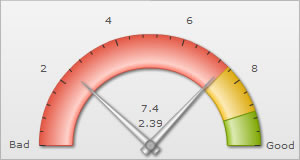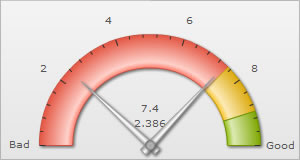No number formatting set - the numbers are automatically rounded off to 2 decimal places Number formatting set to show up to 3 decimal places - hence none of the numbers are rounded off

Note that even though decimals has been set to 3, FusionWidgets XT doesn't forcefully add 0s to 7.4 and convert it to 7.400 or to any of the other numbers on the chart which originally have less than 3 decimal places. To forcibly add 0s to all these numbers, set <chart ... forceDecimals='1' ...>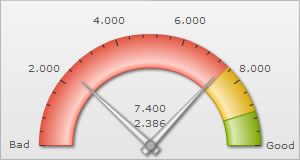Automatic number formatting

FusionWidgets XT allows you to format your numbers by adding K, M and proper commas to the numbers. The commas are added to the numbers by default. But to convert all the big numbers on the chart to numbers made smaller by using K or M, set <chart ...formatNumberScale='1' ...>.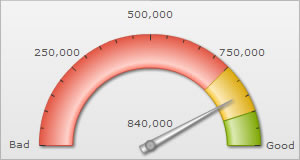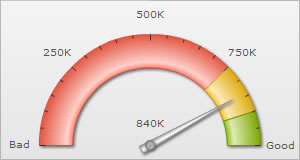No number formatting applied - numbers shown the way they were entered Number formatting applied - numbers converted using K

If you don't want the numbers on the chart to be formatted using commas, you can set <chart .... formatNumber='0' ..> . But, setting formatNumber='0' will not format any decimal places too (even if explicitly specified in XML/JSON).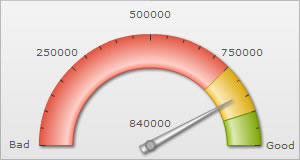Commas removed from all the numbers

Setting custom thousand and decimal separator character

By default, FusionWidgets XT uses . (dot) as decimal separator and , (comma) as thousand separator character. However, you can customize this character depending on your requirements.

To do so, use the decimalSeparator and thousandSeparator attribute. For example, let's set our thousands separator as dot and decimal separator as comma. To do so, you'll have to use the following XML:

<chart ... decimalSeparator=',' thousandSeparator='.' >

Shown below is the output.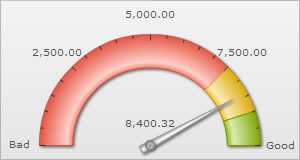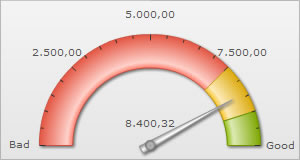Using , as thousand separator and . as decimal separator (default) Using . as thousand separator and , as decimal separator

Configuring thousand separator position

FusionWidgets XT automatically decides the position of the thousand separator. By default, the thousand separator is placed after every three digits from right. A chart with default thousand separator position will look as under: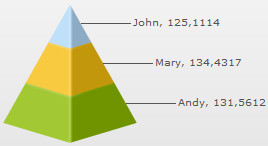FusionWidgets XT allows you to customize the position of the thousand separator and place it as required. To place the thousand separator in custom positions you need to use the thousandSeparatorPosition attribute. Shown below is a chart with the thousand separator placed after four digits from right: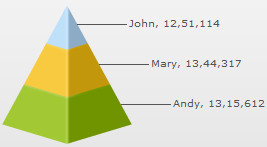In the above chart, the attribute thousandSeparatorPosition is set to '4'. So, the thousand separator (,) is placed after every four digits starting from right.

You can also set multiple values (separated by comma) to this attribute, instead of a single value. Thus, you can specify complex thousand separator positions. For example, specifying multiple values, you can display numbers in Indian currency format, where the first thousand separator (for thousand) is placed after three digits from right and then onwards the thousand separator is placed after every two digits. Shown below is an example chart displaying Indian currency format: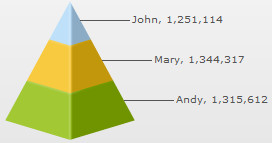In the above chart, we have set thousandSeparatorPosition='2,3'. So, the thousand separator is first placed after 3 digits starting from right and then onwards after every two digits. Given below are some sample combinations of values which can be set to this attribute:

 Value set Description Example thousandSeparatorPosition="0" When this attribute is set to 0, it takes the default value, which is 3, and places the separator after every three digits starting from right. Rule: When zero is specified as the thousand separator position, it assumes the default value, which is 3. 1,344,317,518 thousandSeparatorPosition="2" When this attribute is set to 2, the thousand separator is placed after every two digits starting from right. 13,44,31,75,18 thousandSeparatorPosition="4" When this attribute is set to 4, the thousand separator is placed after every four digits starting from right. 13,4431,7518 thousandSeparatorPosition="1,2" In this case, first the thousand separator is placed after two digits from right. Then onwards the separator will be placed after every digit. 1,3,4,4,3,1,7,5,18 thousandSeparatorPosition="1,2,3" In this scenario, first the separator will be placed after three digits from right, then the separator will be placed after two digits and then onwards it will be placed after every digit. 1,3,4,4,3,17,518 thousandSeparatorPosition="3,1,0" In this scenario, the value 0 will be replaced by the default value 3. So, the thousand separator will be first placed after three digits from right. Then the separator is placed after one digit. Then onwards it will be placed after every three digits. Rule: When zero is specified as the first thousand separator position in a comma separated list of thousand separator positions, it assumes the default value, which is 3. 134,431,7,518 thousandSeparatorPosition="1,0,2" This is a special case where 0 is used as the second value. In this scenario, first the thousand separator is placed after two digits. Next, the value 0 assumes the previous value, which is 2. Hence, the separator will again be placed after two digits and then onwards it will be placed after every digit. Rule: When zero is specified NOT as the first thousand separator position in a comma separated list of thousand separator positions, it assumes the previous value from the list. 1,3,4,4,3,1,75,18 thousandSeparatorPosition="0,1,3" In this scenario, first the thousand separator is placed after three digits. Then it is placed after every digit. Now, the value 0 assumes the previous value, 1 and rest of the separators are placed after every digit. Rule: When zero is specified NOT as the first thousand separator position in a comma separated list of thousand separator positions, it assumes the previous value from the list. 1,3,4,4,3,1,7,518

Note: Any negative value set to this attribute will be converted to a positive value. For example, thousandSeparator="-2" will be converted to thousandSeparator="2".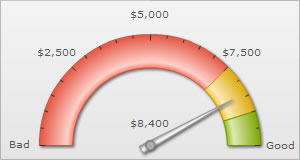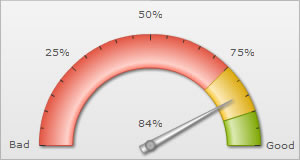\$ set as number prefix % set as number suffix